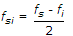11.

Which are the popular IF frequencies for microwave and radar receivers operating on frequencies in the range 1 to 10 GHz

 A. 30, 60 and 70 kHz B. 3, 6 and 7 kHz C. 30, 60 and 70 MHz D. 3, 6 and 7 GHz

Explanation:

No answer description available for this question. Let us discuss.

12.

In a ratio detector

 A. the linearity is worse than in a phase discriminator B. the output is twice that obtainable from a similar phase discriminator C. stabilization against signal strength variations is provided D. the circuit is the same as in a discriminator, except that the diodes are reversed

Explanation:

No answer description available for this question. Let us discuss.

13.

Neutralization is used in RF amplifiers to

 A. stop oscillation B. increase bandwidth C. improve selectivity D. all of the above

Explanation:

No answer description available for this question. Let us discuss.

14.

When fs is the signal frequency and fi is the intermediate frequency, then image frequency fsi is given by

 A. fsi = fs - 2fi B. fsi = fs + 2fi C.D.Explanation:

No answer description available for this question. Let us discuss.

15.

It is known that noise phase modulates the FM wave. As the noise side band frequency approaches the carrier frequency, the noise amplitude

 A. will increase B. will decrease C. will remain constant D. will reduce to negligible value